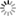##### Personal tools
You are here: Home Bimathematics Research

# Biomathematics Research

### - Mathematical Biology

Mathematical and theoretical biology, or biomathematics, is that branch of biology that uses theoretical analysis, mathematical models, and abstractions of living organisms to study the principles governing the structure, development, and behavior of systems, as opposed to experimental biology, which deals with conducting Experiments to prove and verify scientific theories. The field is sometimes called mathematical biology or biomathematics to emphasize the mathematical aspects, or theoretical biology to emphasize the biological aspects. Theoretical biology focuses more on the development of biological theoretical principles, while mathematical biology focuses on the study of biological systems using mathematical tools, although the two terms are sometimes used interchangeably.

Mathematical biology aims at the mathematical representation and modeling of biological processes using techniques and tools of applied mathematics. It is useful in both theoretical and practical research. Describing systems in a quantitative way means that their behavior can be better modeled, so properties that may not be apparent to the experimenter can be predicted. This requires precise mathematical models.
Due to the complexity of living systems, theoretical biology employs multiple fields of mathematics and contributes to the development of new technologies.

[More to come ...]

##### Document Actions